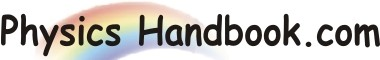HOME TOPICS DEFINITIONS TABLES LAWS INVENTIONS EXPERIMENTS QUIZ VIDEOS
 A B C D E F G H I J K L M N O P Q R S T U V W X Y Z
Kinetic Energy
The kinetic energy of an object is the extra energy which it possesses due to its motion.
It is defined as the work needed to accelerate a body of a given mass from rest to its current velocity.
There are many forms of kinetic energy - vibrational (the energy due to vibrational motion), rotational (the energy due to rotational motion), and translational (the energy due to motion from one location to another).
The following equation is used to represent the kinetic energy (EK) of an object.
EK  =  (1/2) m v2
Where, m  =  mass of object
v  =  speed of object
This equation reveals that the kinetic energy of an object is directly proportional to the square of its speed.
Kinetic energy is a scalar quantity; it does not have a direction.
The standard metric unit of measurement for kinetic energy is the Joule.